Question

A sound wave traveling in 20°C air has a pressure amplitude of 0.448 Pa. What is the intensity of the wave? (Assume the density of air is 1.29 kg/m3. Enter your answer in W/m2.)

________W/m2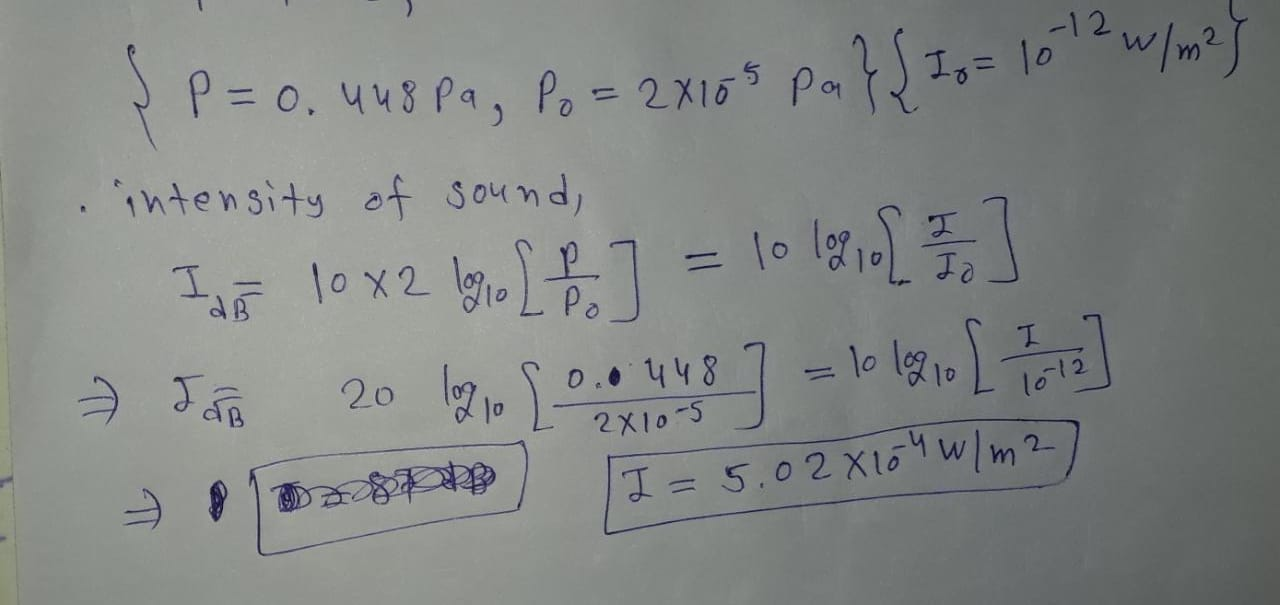#### Earn Coins

Coins can be redeemed for fabulous gifts.

Similar Homework Help Questions
• ### A sound wave in air has a pressure amplitude equal to 4.04 ✕ 10-3 Pa. Calculate...

A sound wave in air has a pressure amplitude equal to 4.04 ✕ 10-3 Pa. Calculate the displacement amplitude of the wave at a frequency of 9.4 kHz. (Note: Use the following values, as needed. The equilibrium density of air is ρ = 1.20 kg/m3; the speed of sound in air is v = 343 m/s. Pressure variations ΔP are measured relative to atmospheric pressure, 1.013 105 Pa.)

• ### 1. An experimenter wishes to generate in air a sound wave that has a displacement amplitude...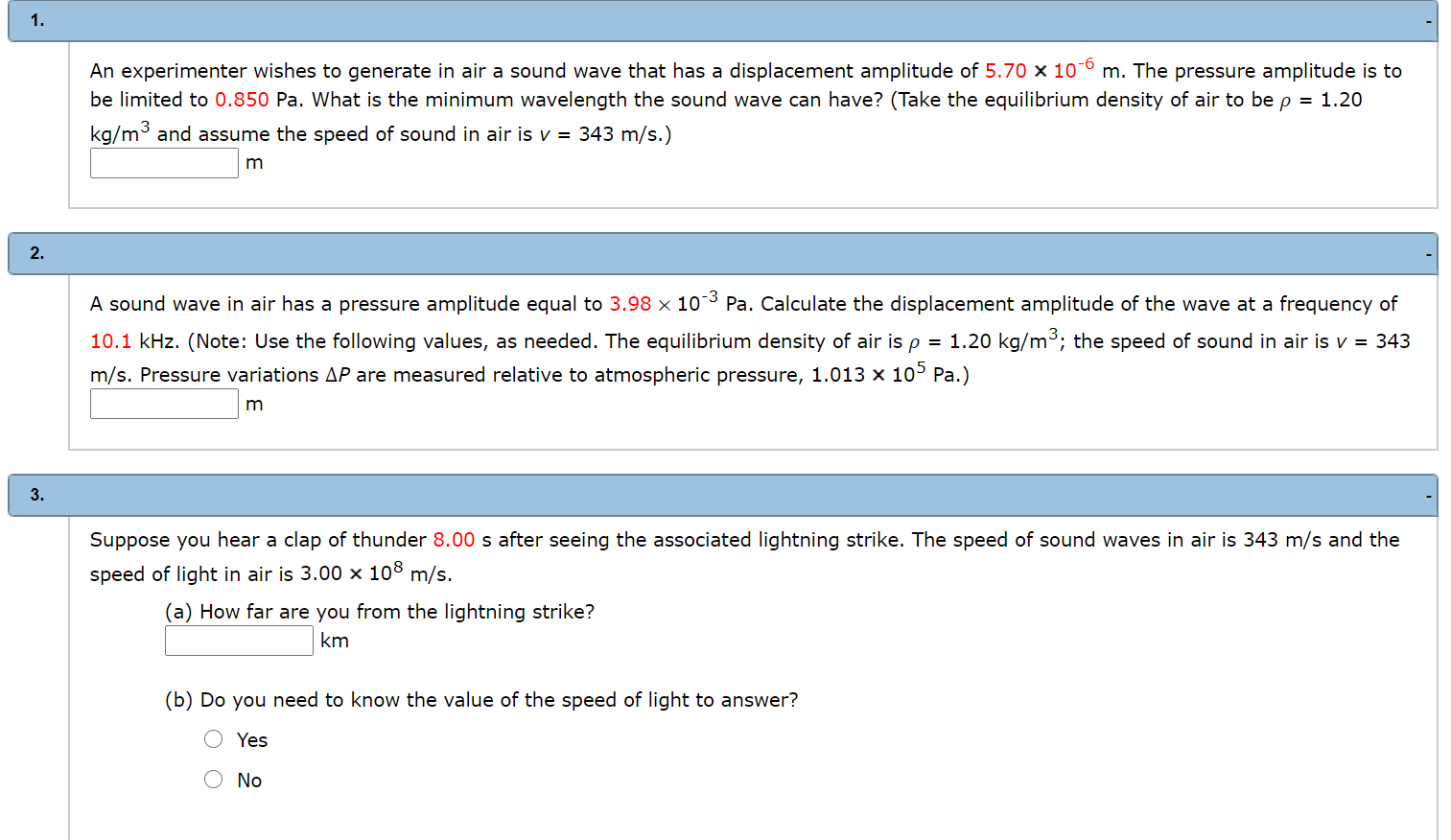1. An experimenter wishes to generate in air a sound wave that has a displacement amplitude of 5.70 x 10-6 m. The pressure amplitude is to be limited to 0.850 Pa. What is the minimum wavelength the sound wave can have? (Take the equilibrium density of air to be p = 1.20 kg/m3 and assume the speed of sound in air is v = 343 m/s.) m 2. A sound wave in air has a pressure amplitude equal to 3.98...

• ### A typical loud sound wave that has a frequency of 1.20 kHz has a pressure amplitude...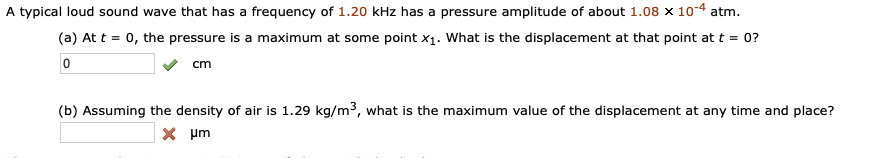A typical loud sound wave that has a frequency of 1.20 kHz has a pressure amplitude of about 1.08 x10-4 atm (a) At t-0, the pressure is a maximum at some point x1. What is the displacement at that point att 07 Cm (b) Assuming the density of air is 1.29 kg/m3, what is the maximum value of the displacement at any time and place?

• ### 4. Consider a sound wave in the air that has displacement amplitude 0.0200 mm. Calculate the...

4. Consider a sound wave in the air that has displacement amplitude 0.0200 mm. Calculate the pressure amplitude for frequencies of (a) 150 Hz; (b) 1500 Hz; (c) 15 000 Hz . In air at normal atmospheric pressure and density, the speed of sound is 344 m/s and the bulk modulus is 1.42×10^5 Pa. Please show all work

• ### The intesity of a wave is I=2π2ρvf2A2, where ρ is the density of the medium, v...

The intesity of a wave is I=2π2ρvf2A2, where ρ is the density of the medium, v is the speed of wave, f is the frequency, and A is the amplitude. The density of air is 1.29 kg/m3, the speed of sound in air is 343 m/s, the intensity of the reference level required to determine the sound level is 1.0×10−12W/m2. What would be the sound level (in dB ) of a sound wave in air that corresponds to a displacement...

• ### A sound has an intensity of 1 W/m2, what is the pressure? 20 Pa O 10...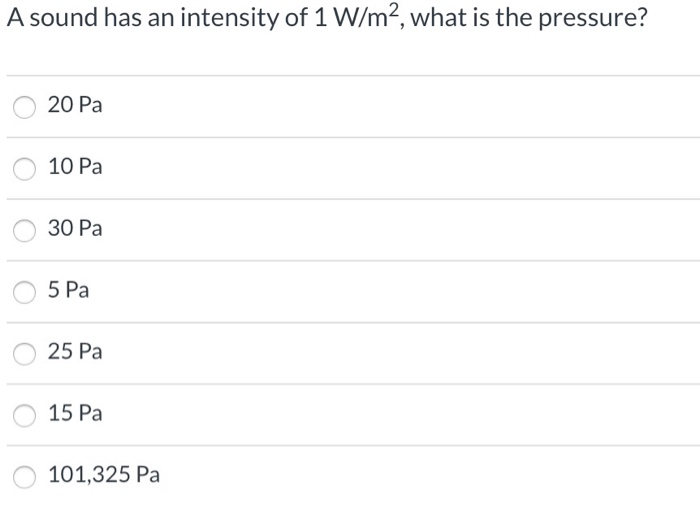A sound has an intensity of 1 W/m2, what is the pressure? 20 Pa O 10 Pa O 30 Pa O 5 Pa 25 Pa 15 Pa 101,325 Pa

• ### Two sound waves (call them X and Y) travel through the air. Wave X has a...

Two sound waves (call them X and Y) travel through the air. Wave X has a wavelength of 0.1 m and a pressure amplitude of 0.01 Pa. Wave Y has a frequency of 3430 Hz and a pressure amplitude of 1 Pa. a.) Which sound wave has a higher pitch? b.) Which sound wave is louder? Fill in the following: The intensity of Y is that of X by ____ times. Soundwave Y is dB soundwave X.

• ### 17. Consider a plane wave at a frequency of 1,000 Hz in air and Lp 87...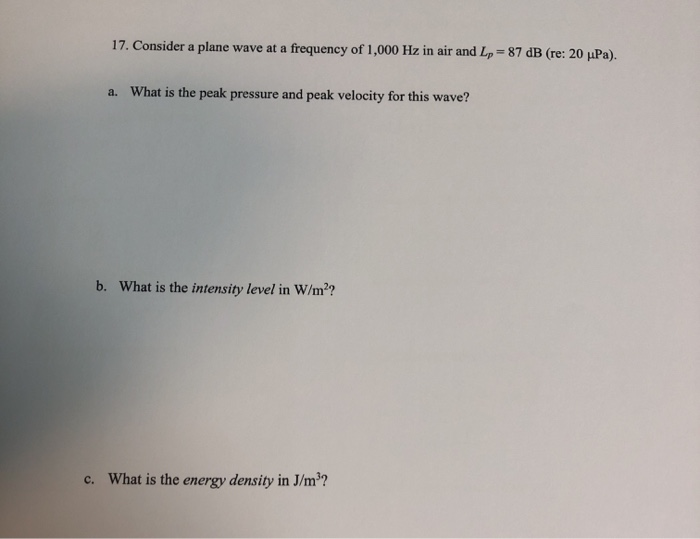17. Consider a plane wave at a frequency of 1,000 Hz in air and Lp 87 dB (re: 20 uPa). What is the peak pressure and peak velocity for this wave? a. What is the intensity level in W/m2? b. What is the energy density in J/m3? c. 17. Consider a plane wave at a frequency of 1,000 Hz in air and Lp 87 dB (re: 20 uPa). What is the peak pressure and peak velocity for this wave? a....

• ### 1. When a sound wave passes through air, and we hear it, the air pressure where we are varies with time. the excess pressure above (and below) atmospheric pressure in a sound wave is given by the...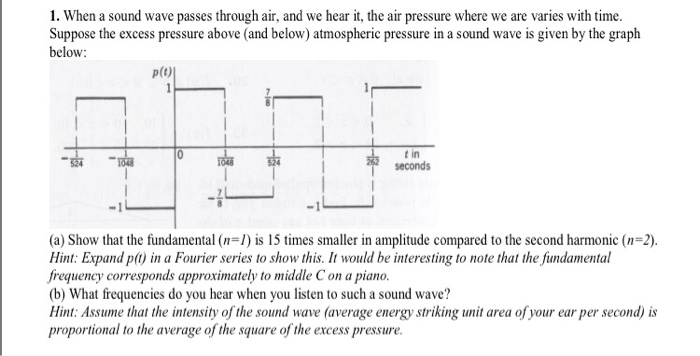1. When a sound wave passes through air, and we hear it, the air pressure where we are varies with time. the excess pressure above (and below) atmospheric pressure in a sound wave is given by the graph below: p(t) t in seconds -1 (a) Show that the fundamental (n ) is 15 times smaller in amplitude compared to the second harmonic (n-2). Hint: Expand p() in a Fourier series to show this. It would be interesting to note that...

• ### 1. A sound has an RMS pressure of 19.7 Pa, Determine the sound pressure level in dB?

1. A sound has an RMS pressure of 19.7 Pa, Determine the sound pressure level in dB?  2. A sound has an intensity of 0.38 W/m2, calculate the SPL in dB?